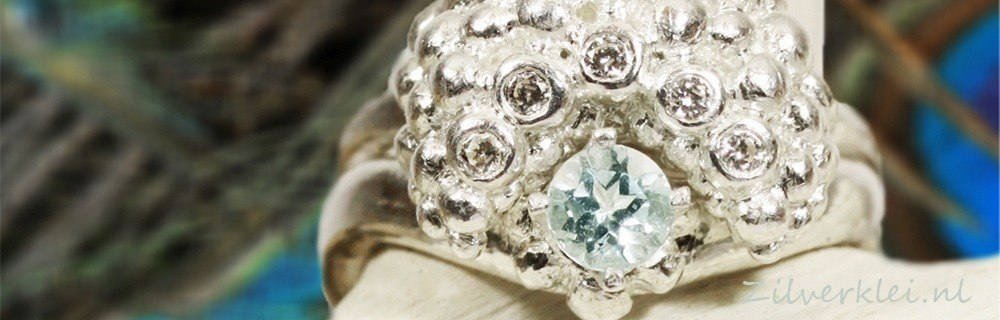# Stones/zirconsThese stones are all heat resistant, if a single stone needs a specific temperature, then you will find this information for the relevant product.

Heat proof

Heat proof

Heat proof

Heat proof

Heat proof

Heat proof

Heat proof

Heat proof

Heat proof

Heat proof

Heat proof

Heat proof

Heat proof

Heat proof

Heat proof

Heat proof

Heat proof

Heat proof

Heat proof

Heat proof

Heat proof

Heat proof

Heat proof

Heat proof

Heat proof

Heat proof

Heat proof

Heat proof

Heat proof

Heat proof

Heat proof

Heat proof

Heat proof

Heat proof

Heat proof

Heat proof

Heat proof

Heat proof

Heat proof

Heat proof

Heat proof

Heat proof

Heat proof

Heat proof

Heat proof

Heat proof

Heat proof

Heat proof

Heat proof

Heat proof